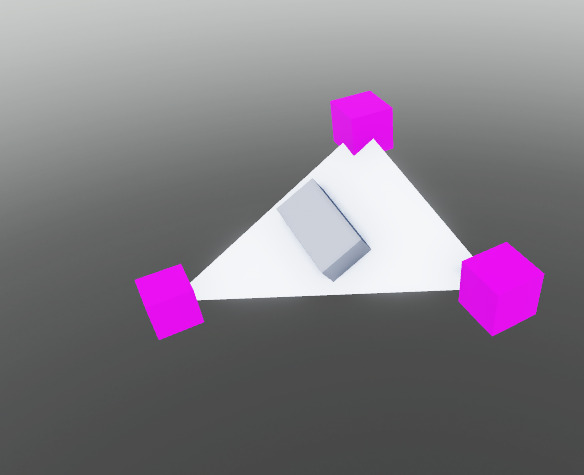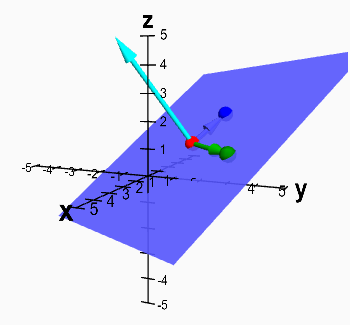# Need help with CFrame calculation

I’ve been trying to make a wave collision using a free module released on devforum:

i’m trying to calculate 3 points and rotate and x object between the 3 points, something like this:but whitout creating the wedges.

what i’ve been tryed:

``````-- this is inside a while true loop
local point1 = workspace.A.Position
local point2 = workspace.B.Position
local point3 = workspace.C.Position

local frontVector = object.CFrame.lookVector
local upVector = object.CFrame.upVector

local cframe = CFrame.new(point1:lerp(point2, tick()/time):lerp(point3, tick()/time))
cframe = cframe * CFrame.fromMatrix(Vector3.new(), frontVector, upVector)
``````

someone can help me with this?

You can get the center position by just averaging out the 3 points (finding the centroid):

``````local pos: Vector3 = (point1+point2+point3) / 3
``````

And the rotation can be derived from the surface normal, which can be derived from cross product.``````local normal: Vector3 = ((point2-point1):Cross(point3-point1)).Unit
``````
``````--have it look at point1 with the UpVector being the normal
local cframe = CFrame.lookAt(pos, point1, normal)
``````

Code used in the example above

``````local part1, part2, part3 = workspace:WaitForChild('p1'), workspace:WaitForChild('p2'), workspace:WaitForChild('p3')
local parent = script.Parent
game:GetService('RunService').Heartbeat:Connect(function()
local p1, p2, p3: Vector3 = part1.Position, part2.Position, part3.Position
local pos: Vector3 = (p1+p2+p3) / 3
local normal: Vector3 = ((p2-p1):Cross(p3-p1)).Unit
parent.CFrame = CFrame.lookAt(pos, p1, normal)
end)
``````
2 Likes

god! thank you for helping me!

This topic was automatically closed 14 days after the last reply. New replies are no longer allowed.